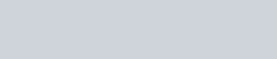Which of the following statements are true and which are false? Give reasons for your answers.

(i) Only one line can pass through a single point.

(ii) There are an infinite number of lines which pass through two distinct points.

(ii) There are an infinite number of lines which pass through two distinct points.

(iv) If two circles are equal, then their radii are equal.

(v) In the following figure, if AB = PQ and PQ = XY, then AB = XY.Solution:

(i) False. Since through a single point, infinite number of lines can pass. In the following figure, it can be seen that there are infinite numbers of lines passing through a single point P.(ii) False. Since through two distinct points, only one line can pass. In the following figure, it can be seen that there is only one single line that can pass through two distinct points P and Q.(iii) True. A terminated line can be produced indefinitely on both the sides.

Let AB be a terminated line. It can be seen that it can be produced indefinitely on both the sides.(iv)True. If two circles are equal, then their centre and circumference will coincide and hence, the radii will also be equal.

(v) True. It is given that AB and XY are two terminated lines and both are equal to a third line PQ. Euclid’s first axiom states that things which are equal to the same thing are equal to one another. Therefore, the lines AB and XY will be equal to each other.